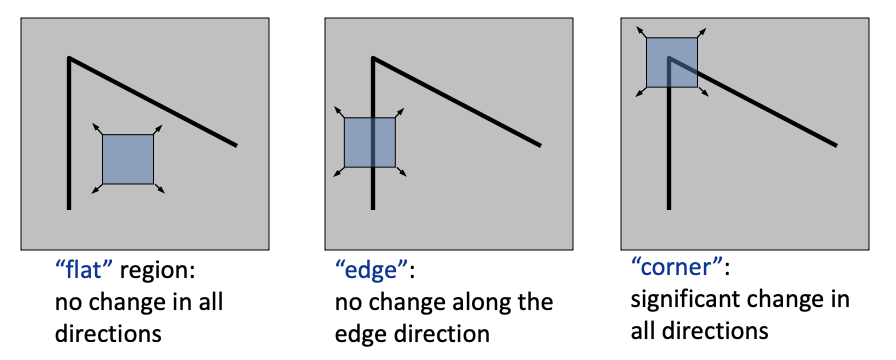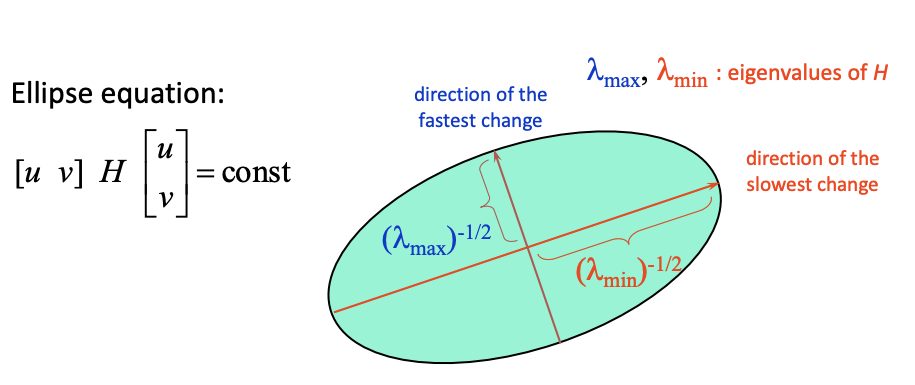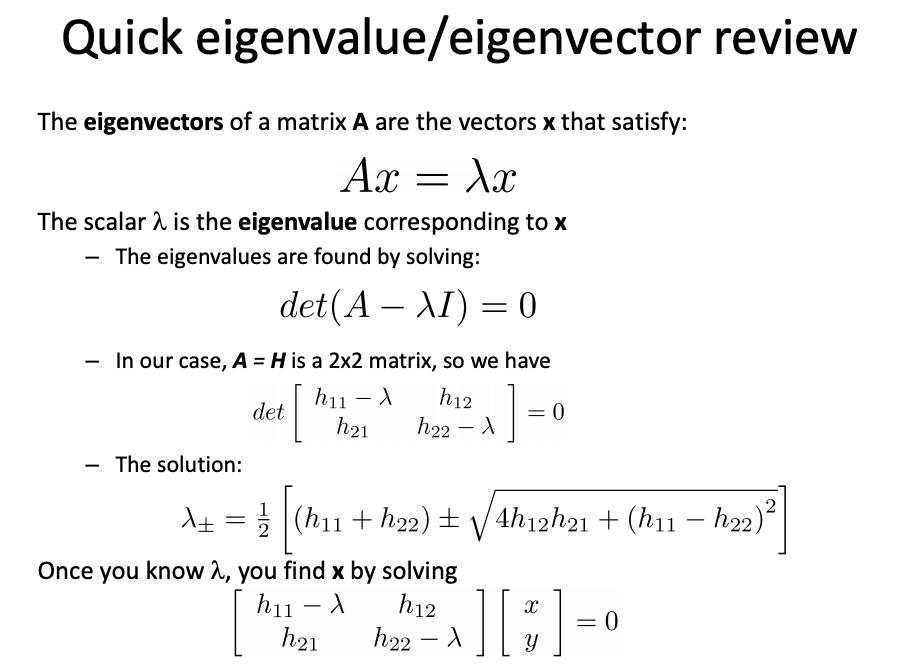# “Feature Detection”

## Key idea

1. Feature detection: find it
2. Feature descriptor: represent it
3. Feature matching: match it

## Harris corner detection:

Suppose we only consider a small window of pixels, corner is a good candidate for featureConsider shifting the window $W$ by $(u,v)$, following formulla defiend SSD:

[\begin{align} E(u, v)=\sum_{(x, y) \in W}[I(x+u, y+v)-I(x, y)]^{2} \end{align}]

This is actually Slow to compute exactly for each pixel and each offset $(u,v)$ but according to Taylor Series expansion, If the motion $(u,v)$ is small, then first order approximation is good
\begin{align*} I(x+u, y+v) & \approx I(x, y)+\frac{\partial I}{\partial x} u+\frac{\partial I}{\partial y} v \approx I(x, y)+\left[I_{x} I_{y}\right] \left[ \begin{array}{c}{u} {v}\end{array}\right] \end{align*}
put it back together:

[\begin{align} {E(u, v)=\sum_{(x, y) \in W}[I(x+u, y+v)-I(x, y)]^{2}\\approx \sum_{(x, y) \in W}\left[I(x, y)+I_{x} u+I_{y} v-I(x, y)\right]^{2} \approx \sum_{(x, y) \in W}\left[I_{x} u+I_{y} v\right]^{2}} \ {E(u, v) \approx \sum_{(x, y) \in W}\left[I_{x} u+I_{y} v\right]^{2} \approx A u^{2}+2 B u v+C v^{2}} \end{align}]

We have \begin{align*} A=\sum_{(x, y) \in W} I_{x}^{2} \end{align*}, \begin{align*} B=\sum_{(x, y) \in W} I_{x} I_{y} \end{align*}, \begin{align*} C=\sum_{(x, y) \in W} I_{y}^{2} \end{align*}

## The second moment matrix

The surface $E(u,v)$ is locally approximated by a quadratic form.

[\begin{align} E(u, v) \approx A u^{2}+2 B u v+C v^{2} \end{align}]

[\begin{align} \approx \left[ \begin{array}{ll}{u} & {v}\end{array}\right] \left[ \begin{array}{ll}{A} & {B} \ {B} & {C}\end{array}\right] \left[ \begin{array}{l}{u} \ {v}\end{array}\right] \end{align}]

\begin{align*} \underbrace{\left[ \begin{array}{ll}{A} & {B} \\ {B} & {C}\end{array}\right]}_{H} \end{align*}

We can visualize H as an ellipse with axis lengths determined by the eigenvalues of H and orientation determined by the eigenvectors of H. The entire function are actually a function of ellipse## eigenvalue/eigenvector review## Corner Detection:

Eigenvalues and eigenvectors of H

• Define shift directions with the smallest and largest change in error
• $x_{max}$ = direction of largest increase in $E$
• $\lambda_{max}$ = amount of increase in direction $x_{max}$
• $x_{min}$ = direction of smallest increase in $E$
• $\lambda_{min}$ = amount of increase in direction $x_{min}$

## Summary

Here’s what you do

• Compute the gradient at each point in the image
• Create the H matrix from the entries in the gradient
• Compute the eigenvalues.
• Find points with large response ($\lambda_{min}$ > threshold)
• Choose those points where $\lambda_{min}$ is a local maximum as features

## The Harris operator

$\lambda_{min}$ is a variant of the “Harris operator” for feature detection

[\begin{align} {f=\frac{\lambda_{1} \lambda_{2}}{\lambda_{1}+\lambda_{2}}} \ {=\frac{\text {determinant}(H)}{\operatorname{trace}(H)}} \end{align}]

Very similar to $\lambda_{min}$ but less expensive (no square root)## YONG HUANG

Hi, I'm Yong Huang. I've recently graduated from Cornell Tech and obtained my master's degree, I shall start my Ph.D. in Computer Science this fall at UC Irvine. Thank you for visiting my site.